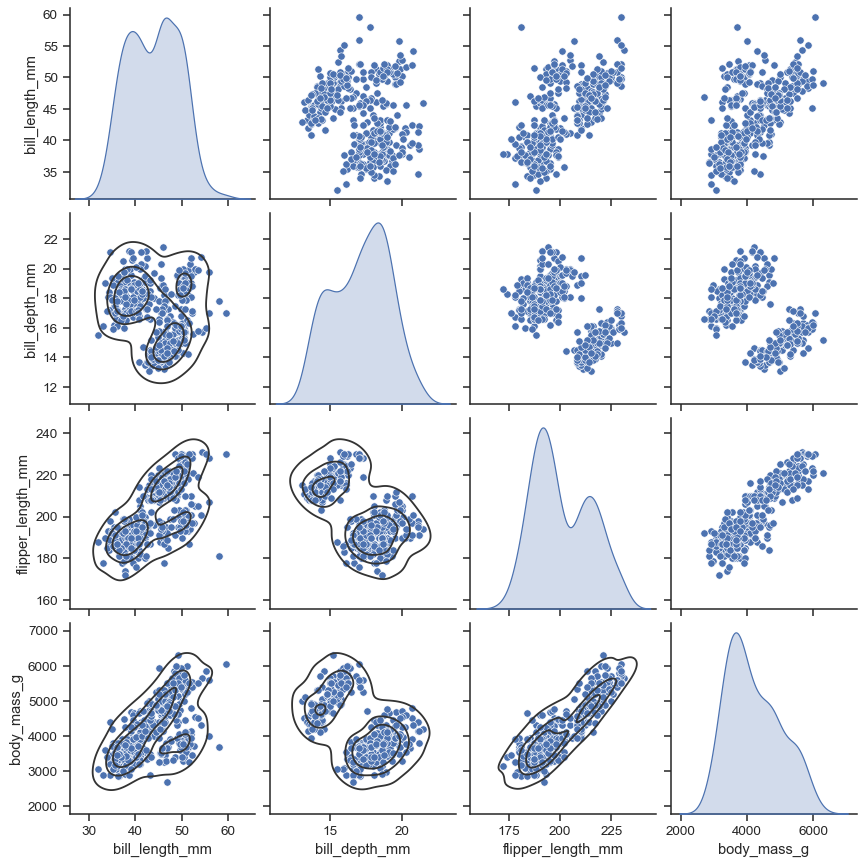# seaborn.pairplot#

seaborn.pairplot(data, *, hue=None, hue_order=None, palette=None, vars=None, x_vars=None, y_vars=None, kind='scatter', diag_kind='auto', markers=None, height=2.5, aspect=1, corner=False, dropna=False, plot_kws=None, diag_kws=None, grid_kws=None, size=None)#

Plot pairwise relationships in a dataset.

By default, this function will create a grid of Axes such that each numeric variable in `data` will by shared across the y-axes across a single row and the x-axes across a single column. The diagonal plots are treated differently: a univariate distribution plot is drawn to show the marginal distribution of the data in each column.

It is also possible to show a subset of variables or plot different variables on the rows and columns.

This is a high-level interface for `PairGrid` that is intended to make it easy to draw a few common styles. You should use `PairGrid` directly if you need more flexibility.

Parameters:
data`pandas.DataFrame`

Tidy (long-form) dataframe where each column is a variable and each row is an observation.

huename of variable in `data`

Variable in `data` to map plot aspects to different colors.

hue_orderlist of strings

Order for the levels of the hue variable in the palette

palettedict or seaborn color palette

Set of colors for mapping the `hue` variable. If a dict, keys should be values in the `hue` variable.

varslist of variable names

Variables within `data` to use, otherwise use every column with a numeric datatype.

{x, y}_varslists of variable names

Variables within `data` to use separately for the rows and columns of the figure; i.e. to make a non-square plot.

kind{‘scatter’, ‘kde’, ‘hist’, ‘reg’}

Kind of plot to make.

diag_kind{‘auto’, ‘hist’, ‘kde’, None}

Kind of plot for the diagonal subplots. If ‘auto’, choose based on whether or not `hue` is used.

markerssingle matplotlib marker code or list

Either the marker to use for all scatterplot points or a list of markers with a length the same as the number of levels in the hue variable so that differently colored points will also have different scatterplot markers.

heightscalar

Height (in inches) of each facet.

aspectscalar

Aspect * height gives the width (in inches) of each facet.

cornerbool

If True, don’t add axes to the upper (off-diagonal) triangle of the grid, making this a “corner” plot.

dropnaboolean

Drop missing values from the data before plotting.

{plot, diag, grid}_kwsdicts

Dictionaries of keyword arguments. `plot_kws` are passed to the bivariate plotting function, `diag_kws` are passed to the univariate plotting function, and `grid_kws` are passed to the `PairGrid` constructor.

Returns:
grid`PairGrid`

Returns the underlying `PairGrid` instance for further tweaking.

`PairGrid`

Subplot grid for more flexible plotting of pairwise relationships.

`JointGrid`

Grid for plotting joint and marginal distributions of two variables.

Examples

The simplest invocation uses `scatterplot()` for each pairing of the variables and `histplot()` for the marginal plots along the diagonal:

```penguins = sns.load_dataset("penguins")
sns.pairplot(penguins)
```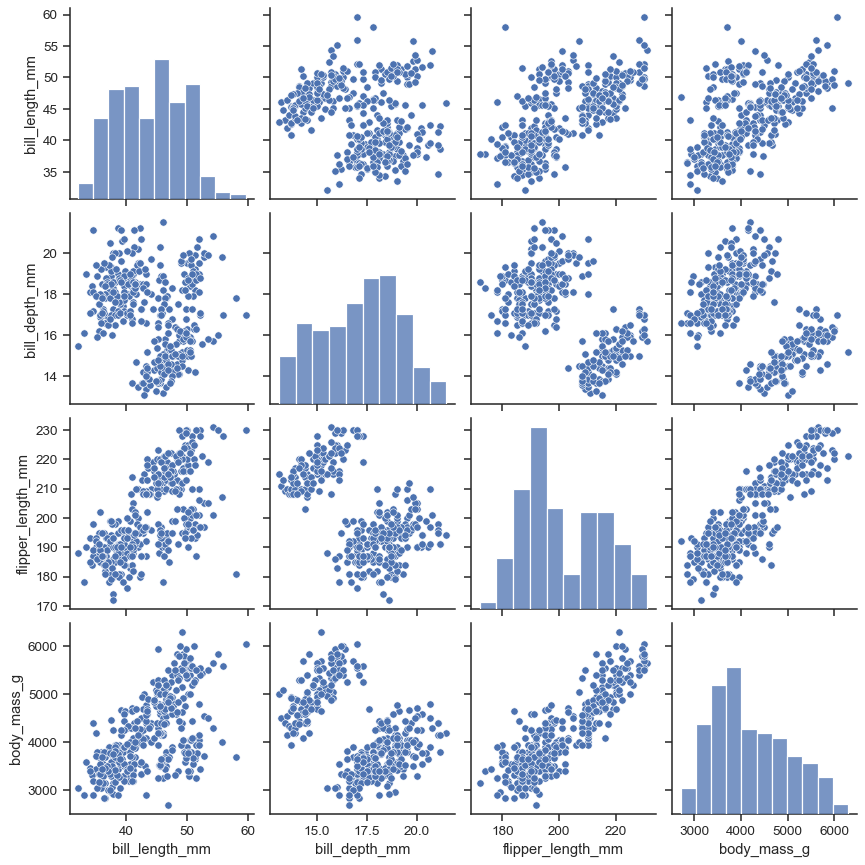Assigning a `hue` variable adds a semantic mapping and changes the default marginal plot to a layered kernel density estimate (KDE):

```sns.pairplot(penguins, hue="species")
```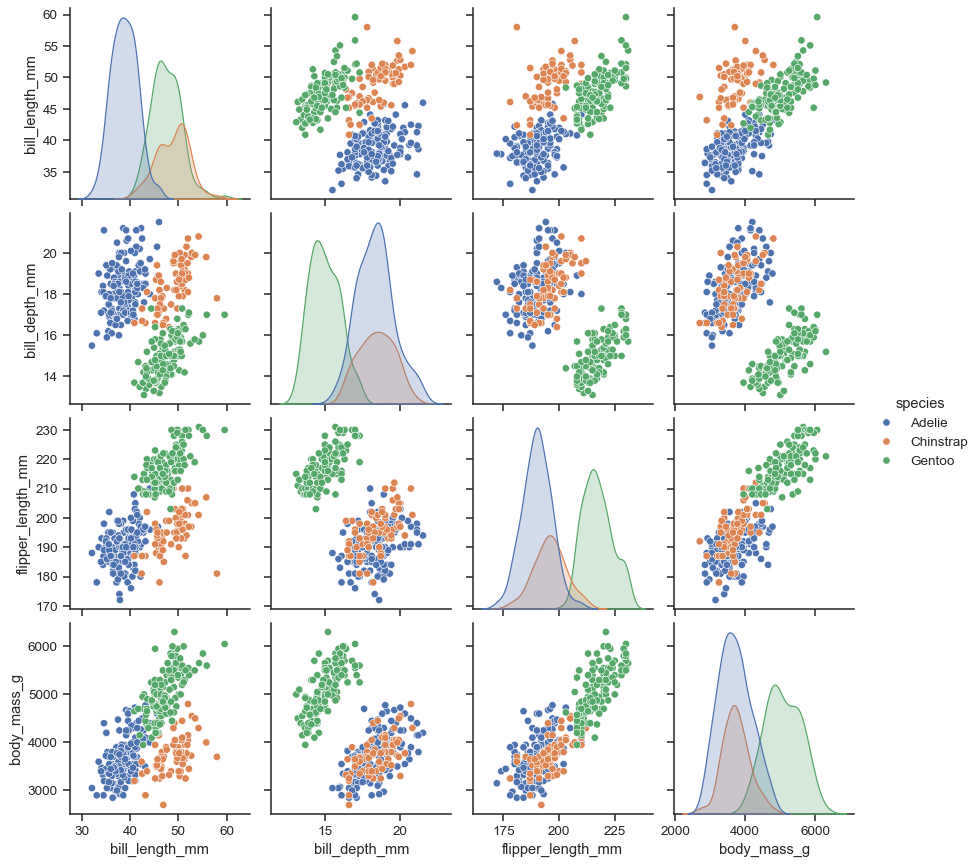It’s possible to force marginal histograms:

```sns.pairplot(penguins, hue="species", diag_kind="hist")
```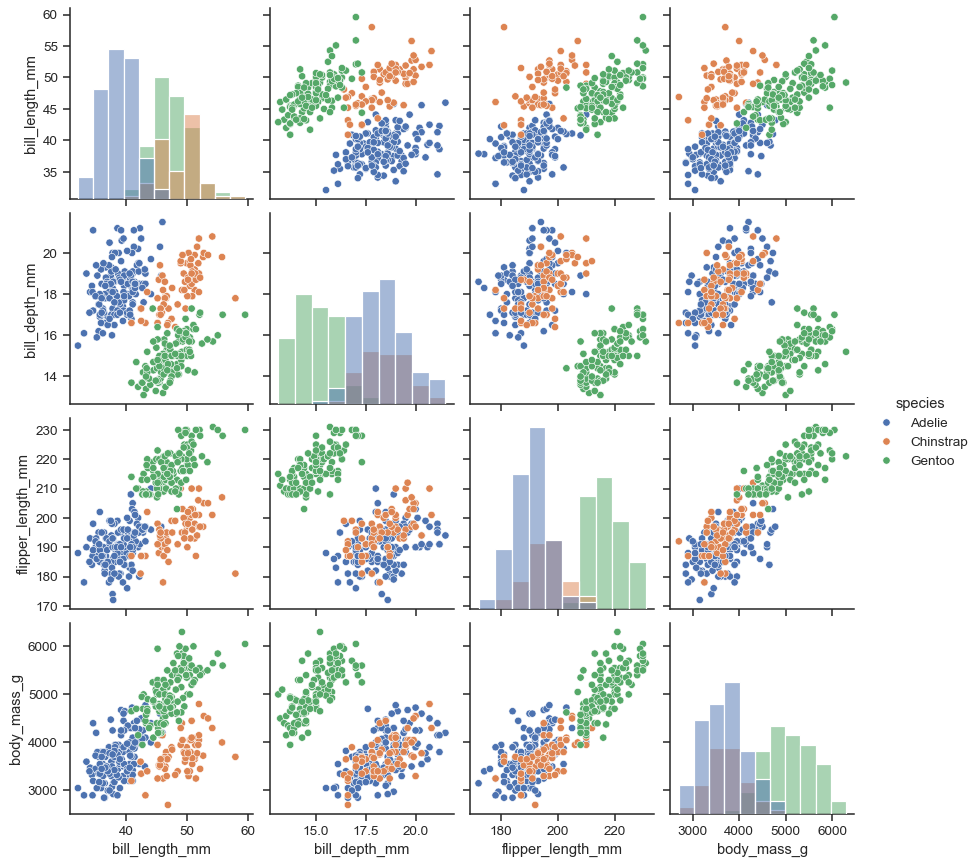The `kind` parameter determines both the diagonal and off-diagonal plotting style. Several options are available, including using `kdeplot()` to draw KDEs:

```sns.pairplot(penguins, kind="kde")
```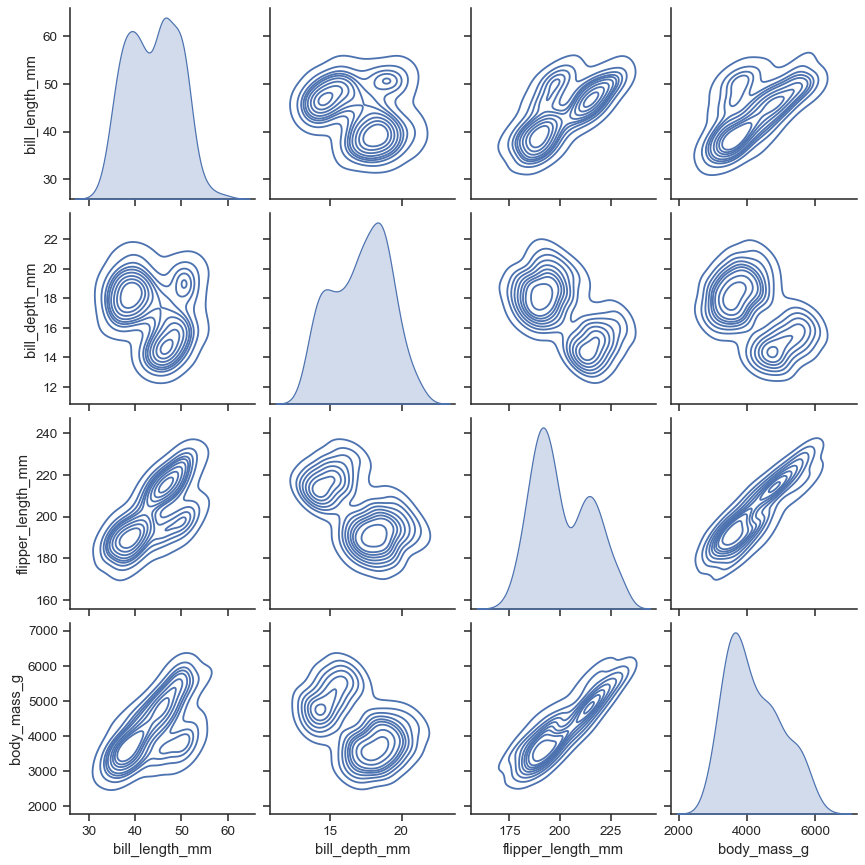Or `histplot()` to draw both bivariate and univariate histograms:

```sns.pairplot(penguins, kind="hist")
```The `markers` parameter applies a style mapping on the off-diagonal axes. Currently, it will be redundant with the `hue` variable:

```sns.pairplot(penguins, hue="species", markers=["o", "s", "D"])
```As with other figure-level functions, the size of the figure is controlled by setting the `height` of each individual subplot:

```sns.pairplot(penguins, height=1.5)
```Use `vars` or `x_vars` and `y_vars` to select the variables to plot:

```sns.pairplot(
penguins,
x_vars=["bill_length_mm", "bill_depth_mm", "flipper_length_mm"],
y_vars=["bill_length_mm", "bill_depth_mm"],
)
```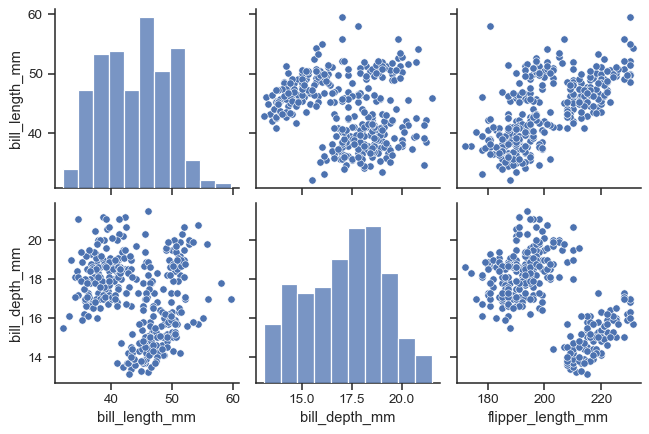Set `corner=True` to plot only the lower triangle:

```sns.pairplot(penguins, corner=True)
```The `plot_kws` and `diag_kws` parameters accept dicts of keyword arguments to customize the off-diagonal and diagonal plots, respectively:

```sns.pairplot(
penguins,
plot_kws=dict(marker="+", linewidth=1),
diag_kws=dict(fill=False),
)
```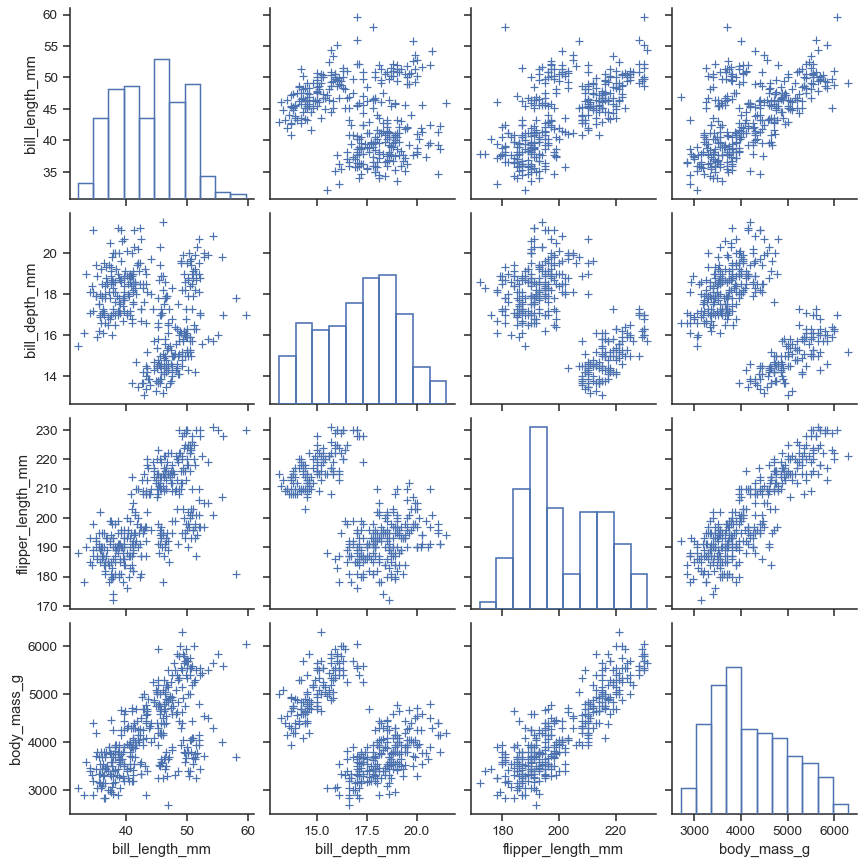The return object is the underlying `PairGrid`, which can be used to further customize the plot:

```g = sns.pairplot(penguins, diag_kind="kde")
g.map_lower(sns.kdeplot, levels=4, color=".2")
```